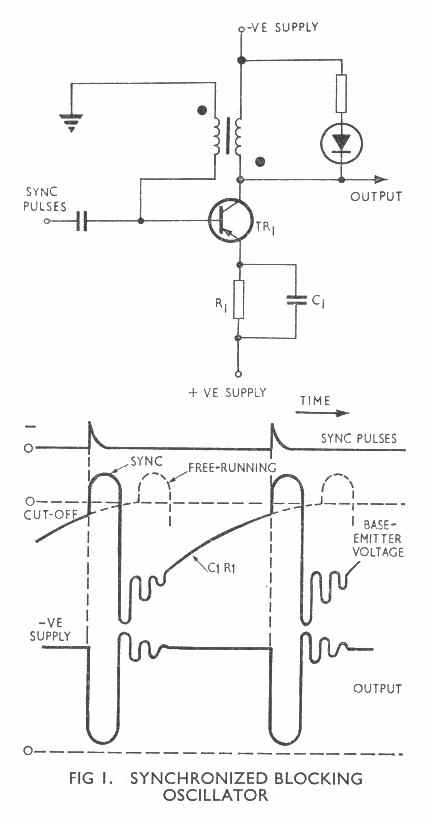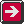Main Radar Home Site Map Radar theory Home AP3302 Pt3 Contents AP3302 Pt3 Section 2Contents AP 3302 Pt. 3 Section 2 CHAPTER 11 Frequency-Dividing & Counting Circuits Introduction Frequency-dividing and counting circuits are both used in radar equipments. In frequency-dividing circuits (sometimes referred to as 'count-down' circuits) one output pulse is obtained for every n input pulses, where n may be any whole number between one and about ten.The frequency divider thus produces an output which is an exact sub-multiple of the pulse train which causes the circuit action. As we shall see, this has many applications in radar. A counting circuit is normally used to provide an output which is proportional to the number of input pulses applied to it. This too has many applications in radar. We shall consider both types of circuit in this chapter. Frequency Division We know from previous work that both the astable multivibrator and the free-running blocking oscillator may be synchronized by applying appropriate sync pulses to the control grid (valve version) or to the base-emitter circuit (transistor version). The p.r.f. of the sync pulses must be slightly higher than that of the free-running circuit (Fig 1) and their amplitude must be such that they lift the circuit into conduction before it would normally cut on. The output is then locked to the sync pulses. Frequency division is merely an extension of this idea. The p.r.f. of the input pulses is now made much higher than that of the free-running circuit and the circuit constants are then adjusted such that, say, every third input pulse brings the circuit prematurely into conduction. In this case one output pulse is produced for every three input pulses and the circuit is said to have a count-down ratio of 3 :1. Count-down ratios of up to about 10:1 may be obtained in this way. Application of Frequency Division Frequency dividers have many applications. We shall consider only one at this stage. We saw in an earlier chapter that cal pips may be used to calibrate a type A display. A display with large cal pips at ten-mile intervals and small cal pips at five-mile intervals may be required. A cal pip generator, consisting of a triggered blocking oscillator, may be used to provide the five-mile cal pips (Fig 2). The output from this is applied to the display and also as the input to a frequency divider. This may be a free-running blocking oscillator so adjusted that every second input pulse from the cal pip generator brings the divider into conduction slightly earlier than normal. The frequency divider therefore produces one output pulse for every two input pulses so that its frequency is half that of the input. This circuit therefore produces ten-mile cal pips.Previous page To top of this page Next Page

Constructed by Dick Barrett
Email: